﻿﻿ Magnetic Field of Transformerexamples｜products｜Murata Software Co., Ltd.# Example14Magnetic Field of Transformer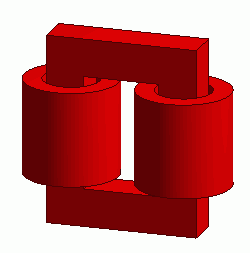General

• Two coils united by loop magnetic core are solved.

• The coupling coefficient, the vectors of the magnetic field and the magnetic flux density are solved.

• Unless specified in the list below, the default conditions will be applied.

### Analysis Space

 Item Settings Analysis Space 3D Model unit mm

### Analysis Conditions

 Item Settings Solvers Magnetic Field Analysis [Gauss] Analysis Type Static analysis Options N/A

### Model

Bodies of two loop coils (Coil1, Coil2) and magnetic core are defined.

The body attributes are set so that the currents of two loop coils flow in the opposite direction each other.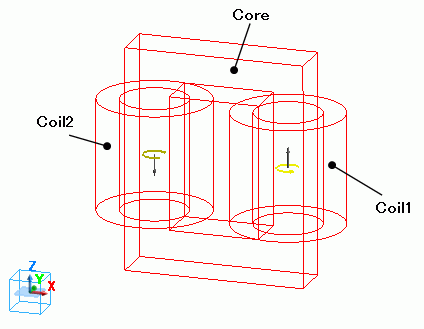### Body Attributes and Materials

 Body Number/Type Body Attribute Name Material Name 1/Solid Coil2 008_Cu * 3/Solid Coil1 008_Cu * 4/Solid Core Core

* Available from the Material DB

The material properties are set up as follows:

 Material Name Tab Properties core Permeability Relative Permeability 5×10^3

Body attribute is set up as follows to apply current to the loop coil.

 Body Attribute Name Tab Settings Coil1 Current Waveform: Constant Current: 1[A] Turns: 100[Turns] Direction: Loop Coil/Magnetic Field Direction: Magnetic Field Vector: X=0, Y=0, Z=1 Coil2 Current Waveform: Constant Current: 1[A] Turns: 100[Turns] Direction: Loop Coil/Magnetic Field Direction: Magnetic Field Vector: X=0, Y=0, Z=-1

No setting.

### Results

To see the results of inductance calculation, go to the [Results] tabclick [Table]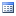.

The inductances of the loop coils are output as follows.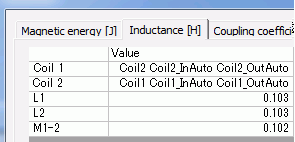The coupling coefficient is output as follows.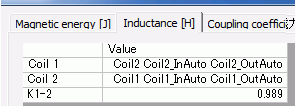The vectors of the magnetic field are shown below.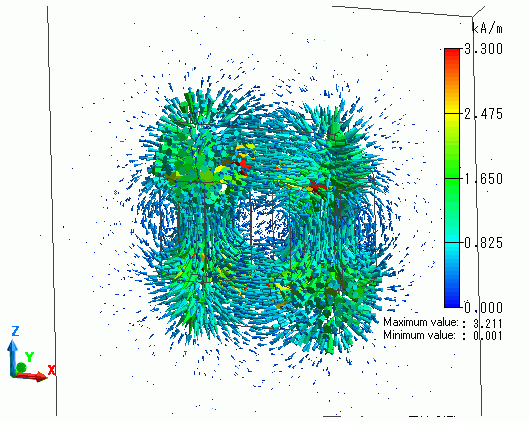The vectors of the magnetic flux density are shown below.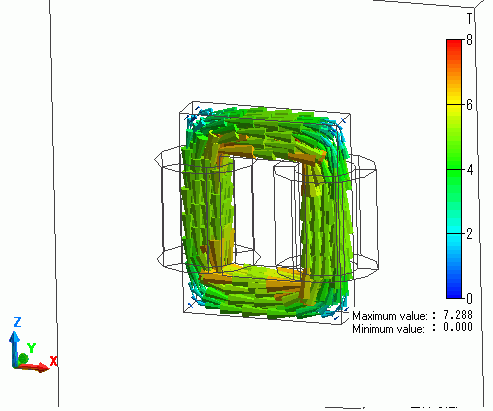The flux density is looping through the iron core.

The vectors of the current density are shown below.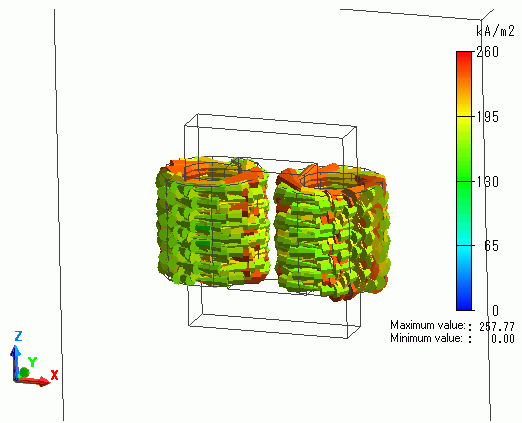﻿ ﻿